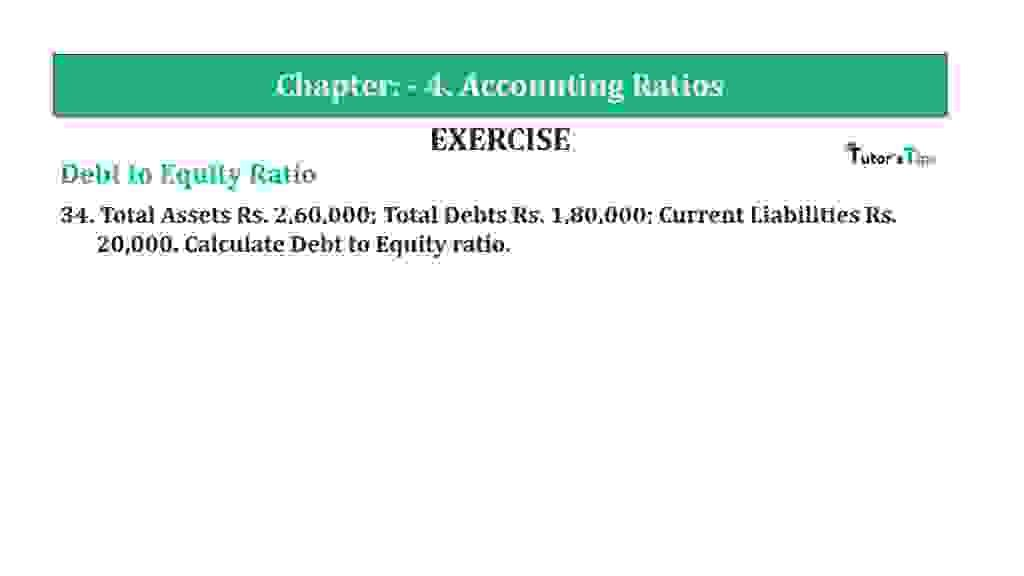# Question 34 Chapter 4 of +2-B – T.S. Grewal 12 ClassQuestion 34 Chapter 4 of +2-B

34. Total Assets Rs. 2,60,000; Total Debts Rs. 1,80,000; Current Liabilities Rs.
20,000. Calculate Debt to Equity ratio.

### The solution of Question 34 Chapter 4 of +2-B: –

 Total Debts = Rs. 1,80,000 Current Liabilities = Rs. 20,000 Equity = Total Assets – Total Liabilities = Rs.2,60,000 – Rs.1,80,000 Equity = Rs.80,000 Long-term Debts = Total Debts – Current Liabilities = Rs.1,80,000 – Rs.20,000 Debt = Rs. 1,60,000

 Debt to Equity Ratio = Long-term Debts = Rs.1,60,000 Equity Rs.80,000 = 2: 1

Balance Sheet: Meaning, Format & Examples

Thanks, Please Like and share with your friends

Comment if you have any question.

Also, Check out the solved question of previous Chapters: –

### T.S. Grewal’s Double Entry Book Keeping (Vol. II: Accounting for Companies)T.S. Grewal’s Analysis of Financial Statements